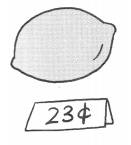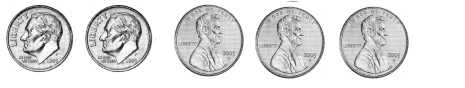# Math in Focus Grade 1 Chapter 19 Practice 1 Answer Key Penny, Nickel, and Dime

Go through the Math in Focus Grade 1 Workbook Answer Key Chapter 19 Practice 1 Penny, Nickel, and Dime to finish your assignments.

## Math in Focus Grade 1 Chapter 19 Practice 1 Answer Key Penny, Nickel, and Dime

Question 1.
Match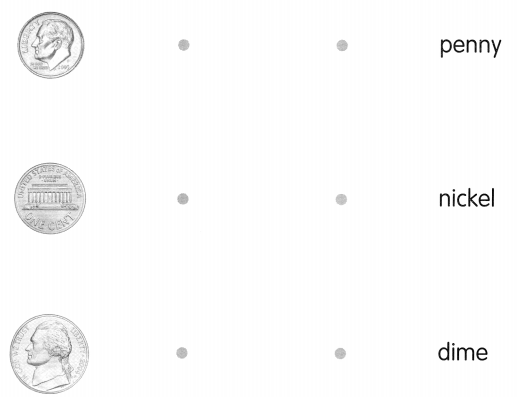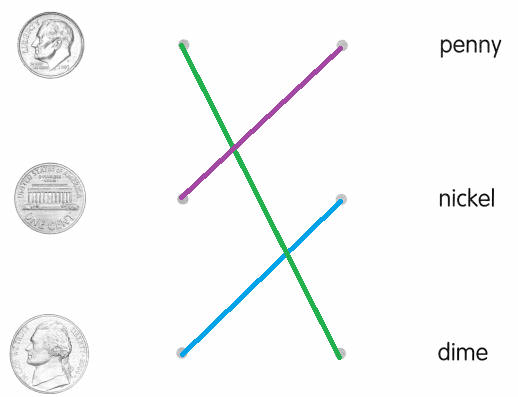Explanation:
The above given coins are the currencies of different countries, especially United States.
1 penny = 1 cent
1 nickel = 5 cents
1 dime = 10 cents

Write the value.

Example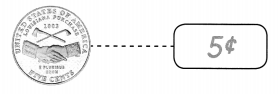Question 2.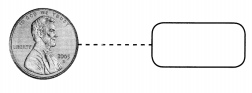1¢, Penny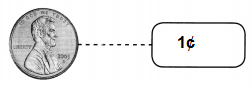Explanation:
One penny (1p) coin is a unit of currency equaling one-hundredth of a pound.

Question 3.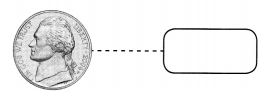5¢, Nickel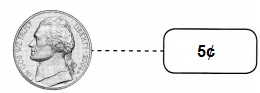Explanation:
Nickel represents to currency.
1 Nickel is equal to 5 cents or pennies.

Question 4.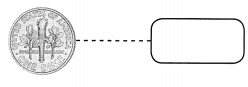Dime 10¢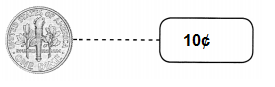Explanation:
Dime represents to currency.
The picture shows the other side of coin.
1 Dime is equal to 10 cents or pennies.

Question 5.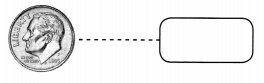Dime 10¢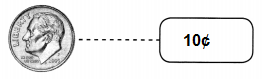Explanation:
Dime represents to currency.
The picture shows the other side of coin.
1 Dime is equal to 10 cents or pennies.

Complete.

Question 6.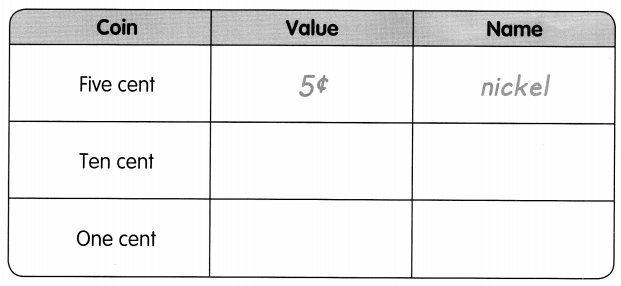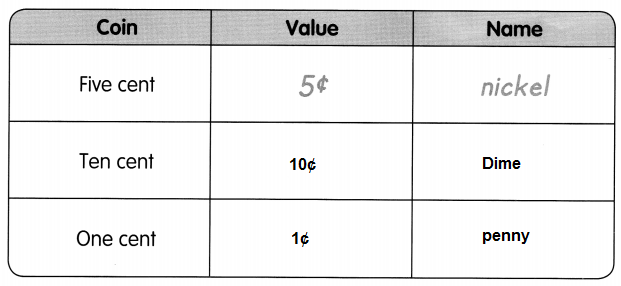Explanation:
The above table shows the coins, their values and their names.
5 cents = 1 nickel
10 cents = 1 dime
1 cent = 1 penny

Keisha has these coins.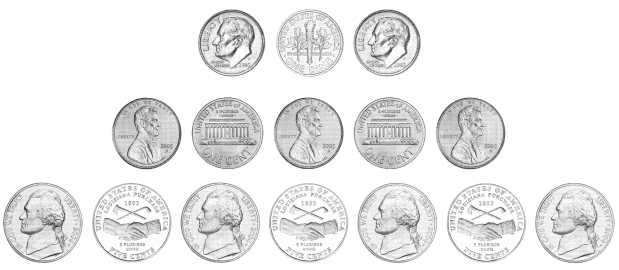Group the pennies, nickels and dimes. Then count on to fill in the blanks.

Question 7.
There are ___________ dimes.
3 dimes
Explanation:
Keisha has a group of coins.
After grouping the coins to pennies, nickels and dimes,
he has 3 dimes in all.

Question 8.
There are ___________ coins in all.
15 coins
Explanation:
Keisha has a group of coins,
with a combination of pennies, nickels and dimes.
Total coins in all are 15.

Question 9.
There are ___________ fewer dimes than nickels.
4 fever dimes then nickels
Explanation:
Keisha has a group of coins.
After grouping the coins to pennies, nickels and dimes.
He has 3 dimes and 7 nickels.
So, There are 4 fewer dimes than nickels.

Question 10.
There are ___________ more pennies than dimes.
2 more pennies than dimes.
Explanation:
Keisha has a group of coins.
After grouping the coins to pennies, nickels and dimes.
He has 5 pennies, 3 dimes and 7 nickels.
So, There are 2 more pennies than dimes.

Find the value.

Question 11.
pennies ___________
5¢ or 5 pennies
Explanation:
1 penny = 1 cent
5 pennies = 5 cents

Question 12.
nickels ___________
Explanation:
1 nickel = 5 cents
7 nickels = 7 x 5¢
= 35¢

Question 13.
dimes ___________
Explanation:
1 dime = 10 cents
3 dimes = 30 x 3
= 30¢

Find the value.

Example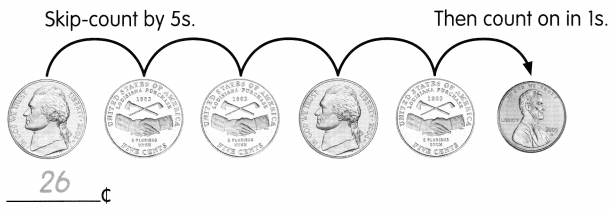Count on from the coin with the greatest value. Count on by 10s for climes. Count on by 5s for nickels. Count on by Is for pennies.

Question 14.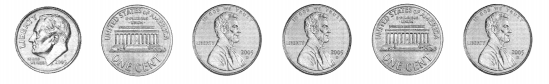___________¢
Explanation:
first coin is dime,
1 dime = 10¢
next 5 coins are cents
5 + 1 + 1 + 1 + 1 + 1 = 10¢

Question 15.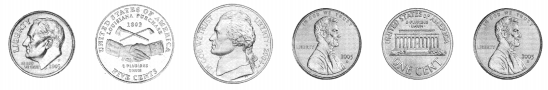___________¢
Explanation:
first coin is dime,
1 dime = 10 cents
next 2 coins are nickels,
1 nickel = 5 cents
next 3 coins are pennies,
1 penny = 1 cent
10 + 5 + 5 + 1 + 1 + 1= 23¢

Solve the riddle. Circle the correct coin.

Example
You need 10 of me to make a dime. What am I?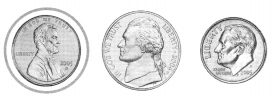Question 16.
I am silver-colored. You need two of me to make ten cents. What am I?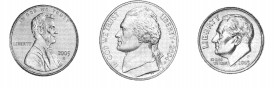Nickel 5¢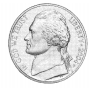Explanation:
Nickel is available in silver color.
1 nickel = 5 cents

Question 17.
Two of me has a value of 20c. What am I?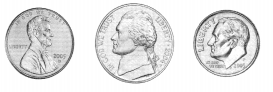Dime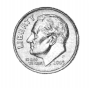Explanation:
1 dime = 10 cents
2 dimes = 20¢

Find how many of each coin are needed. Fill in the blanks.

Question 18.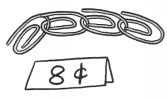___________ dimes, __________ nickels, __________ pennies
1 nickel and 3 pennies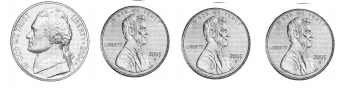Explanation:
1 nickel = 5 cents
1 penny = 1 cent
So, 1 nickel and 3 pennies = 8 cents

Question 19.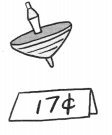___________ dimes, __________ nickels, __________ pennies
One dime, One nickel and 2 pennies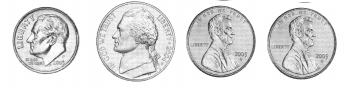Explanation:
1 dime = 10 cents
1 nickel = 5 cents
1 penny = 1 cent
One dime, One nickel and 2 pennies = 17 cents

Question 20.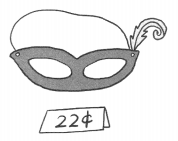___________ dimes, __________ nickels, __________ pennies
Two dimes and two pennies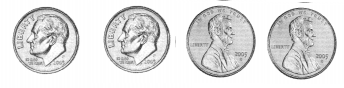Explanation:
1 dime = 10 cents
1 penny = 1 cent
Two dimes and two pennies = 22 cents

Draw the coins to buy each thing. Use pennies 1¢, nickels 5¢ and dimes 10¢.

Question 21.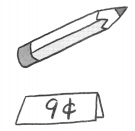5¢ + 4¢ = 9¢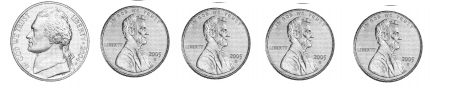Explanation:
1 nickel = 5 cents
1 penny = 1 cent
So, 1 nickel + 4 pennies
5¢ + 4¢ = 9¢

Question 22.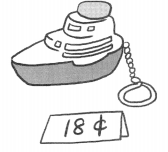10 + 5 + 1 + 1 + 1 = 18¢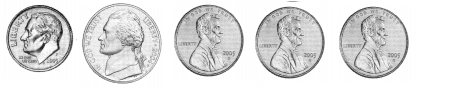Explanation:
1 dime = 10 cents
1 nickel = 5 cents
1 penny = 1 cent
1 dime + 1 nickel + 3 pennies
10 + 5 + 1 + 1 + 1 = 18¢

Question 23.